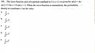# If the wave function is normalized, what is the probability density at x?

hidemi
Homework Statement:
How to know the answer is D?
Relevant Equations:
non
The wave function ψ(x) of a particle confined to 0 ≤ x ≤ L is given by ψ(x) = Ax, ψ(x) = 0 for x < 0 and x > L. When the wave function is normalized, the probability density at coordinate x has the value?

(A) 2x/L^2. (B) 2x^2 / L^2. (C) 2x^2 /L^3. (D) 3x^2 / L^3. (E) 3x^3 / L^3

Ans : D

#### Attachments

•1.jpg
25.9 KB · Views: 54

Homework Helper
Is there a question here ?

Homework Helper
I see you are pretty new here, so!​

And the PF guidelines ask that you post your own attempt at solution. What did you find ?

Homework Helper
Homework Statement:: How to know the answer is D?
Relevant Equations:: non

The wave function ψ(x) of a particle confined to 0 ≤ x ≤ L is given by ψ(x) = Ax, ψ(x) = 0 for x < 0 and x > L. When the wave function is normalized, the probability density at coordinate x has the value?

(A) 2x/L^2. (B) 2x^2 / L^2. (C) 2x^2 /L^3. (D) 3x^2 / L^3. (E) 3x^3 / L^3

Ans : D
Let me help some more: the homework statement is the part in italics.
Your question (which I missed) is the part in red

And the answer follows from calculating A and then the probability density. For that you need the relevant equations. 'non' doesn't do it.

Hint: do the normalizatiion.

##\ ##

hidemi
I see you are pretty new here, so!​

And the PF guidelines ask that you post your own attempt at solution. What did you find ?
I think I got it, thanks!

•BvU